# Line Chart DI for IBPS , SBI , RBI | Quantitative Aptitude | Quiz-1

## Line Chart DI for IBPS , SBI , RBI: Quiz 1

Line Chart DI plays a significant role in Quantitative Aptitude Section of banking exams such as IBPS, SBI and RBI PO and Clerk. You will get at least 4-5 questions from Line Chart DI in one of IBPS, SBI and RBI PO & clerk exam. So, aspirants should focus on Line Chart DI questions in detail. Here, we are providing you with the Line Chart DI questions quiz with the detailed solution so that you can easily prepare for Line Chart DI questions. We are providing here all-important latest pattern-based questions and Previous Year Questions of Line Chart DI of various Government Exam like IBPS, SBI, and RBI PO and Clerk exam. This Line Chart DI quiz we are providing is free. Attempt this Line Chart DI quiz to practice important questions with answers and solutions. And score better in IBPS, SBI and RBI PO and Clerk exam.

Line Chart DI Quiz to improve your Quantitative Aptitude for SBI Po & SBI clerk exam IBPS PO Reasoning , IBPS Clerk Reasoning , IBPS RRB Reasoning, LIC AAO ,LIC Assistant  and other competitive exam

Directions (1-5): Study the following graph carefully to answer these questions:

Populations of two States (in millions) over the years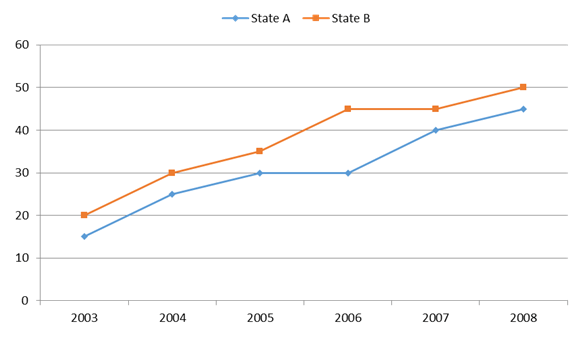1. For state B the per cent rise in population from the previous year was the highest in which of the following years?

(a) 2008

(b) 2006

(c) 2005

(d) 2004

(e) 2007

1. Ans(d)

2004

2. What was the average population of state B (in millions) for all the years together?

(a) 38.5

(b) 28.5

(c) 35

(d) 26

(e) 37.5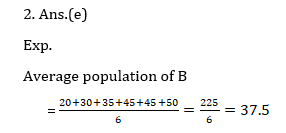3. What is the per cent rise in population of state A in 2007 from the previous year?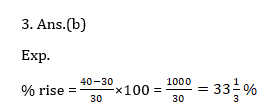4. What is the ratio between the total population of states A and B respectively for all the years together?

(a) 37 : 45

(b) 37 : 43

(c) 43 : 37

(d) 45 : 37

(e) None of these5. Population of state A in 2005 is what per cent of its total population for all the years together?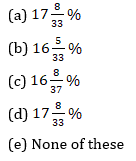Directions (6-10): First line graph shows the Cost Price as a percentage of mark price of a product and second line graph shows the mark price as a percentage with respect to the selling price of a product in five different years from 2001 to 2005.(Hint – Let SP of the product in 2005 = 100x, Then from the second line graph MP = 120x

And, from the first line graph CP = 48x)

6. If the mark price of the product in the year 2002, 2003 and 2005 was same then the CP of the product in year 2002 and 2003 together was what percent more than the cost price of the product in the year 2005?

(a) 100%

(b) 125%

(c) 150%

(d) 200%

(e) 50%7. If the CP of the product in year 2006 was th times of the CP of the product in year 2005 and there was same profit (in Rs.) in year 2006 as in year 2005, then find the selling price (in Rs) of the product in year 2006 if selling price of the product in year 2005 is 100 Rs.

(a) 50 Rs

(b) 60 Rs.

(c) 70 Rs

(d) 40 Rs.

(e) none of these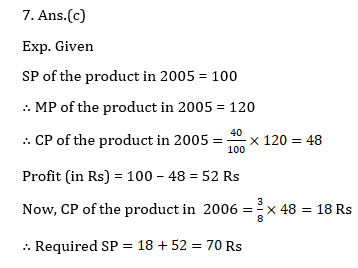8. If the cost price of the product in year 2001, 2002 and 2003 were same then find the ratio of mark price of the product in the same year?

(a) 1:3:2

(b) 3:2:1

(c) 1:2:3

(d) 2:1:3

(e) none of these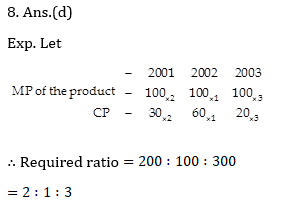9. If the mark price of the product in year 2003 was 1050 Rs and cost price of the product in year 2004 was 700 Rs then find the average selling price of the product in year 2003 and 2004 together?

(a) 560

(b) 350

(c) 450

(d)  800

(e) Rs. 600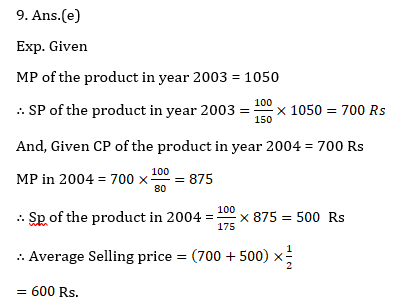10. Total CP of the products in all of the given years together was what % less then the total MP of the product in all of the given years?

(a) 64%

(b) 52%

(c) 62%

(d) 65%

(e) Can’t be determined

10. Ans.(e)

Exp. Since we don’t know exact values of M.P. and C.P. Hence the answer can’t be determined.

#### Attempt Quantitative Aptitude Topic Wise Online Test Series

Recommended PDF’s for :

#### Most important PDF’s for Bank, SSC, Railway and Other Government Exam : Download PDF Now

AATMA-NIRBHAR Series- Static GK/Awareness Practice Ebook PDF Get PDF here
The Banking Awareness 500 MCQs E-book| Bilingual (Hindi + English) Get PDF here
AATMA-NIRBHAR Series- Banking Awareness Practice Ebook PDF Get PDF here
Computer Awareness Capsule 2.O Get PDF here
AATMA-NIRBHAR Series Quantitative Aptitude Topic-Wise PDF Get PDF here
AATMA-NIRBHAR Series Reasoning Topic-Wise PDF Get PDF Here
Memory Based Puzzle E-book | 2016-19 Exams Covered Get PDF here
Caselet Data Interpretation 200 Questions Get PDF here
Puzzle & Seating Arrangement E-Book for BANK PO MAINS (Vol-1) Get PDF here
ARITHMETIC DATA INTERPRETATION 2.O E-book Get PDF here
3